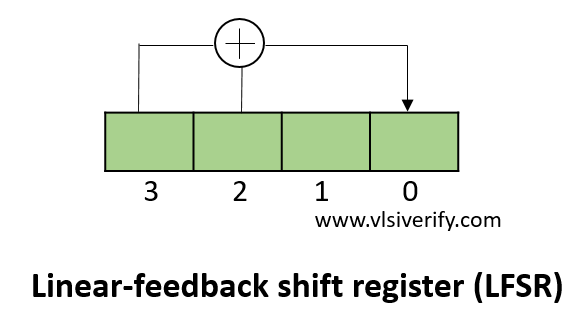Tutorials

A Linear-feedback shift register (LFSR) is another variation of shift register whose input bit is a linear function (typically XOR operation) of its previous state. It is generally used as a pseudo-random number generator, whitening sequence, pseudo-noise sequence, etc.

The bit positions that act as an input to a linear function to affect the next state are known as taps.

## 4-bit pseudo-random sequence generatorAt every step,

1. Q xor Q
2. Q = Q << 1
3. The result of the XOR operation is fed to the LSB (0th bit)

In the above pseudo-random sequence generator, taps are 4 and 3.

## LFSR Verilog Code

``````module LFSR(input clk, rst, output reg [3:0] op);
always@(posedge clk) begin
if(rst) op <= 4'hf;
else op = {op[2:0],(op^op)};
end
endmodule``````

## Testbench Code

``````module TB;
reg clk, rst;
wire [3:0]op;

LFSR lfsr1(clk, rst, op);

initial begin
\$monitor("op=%b",op);
clk = 0; rst = 1;
#5 rst = 0;
#50; \$finish;
end

always #2 clk=~clk;

initial begin
\$dumpfile("dump.vcd"); \$dumpvars;
end
endmodule``````

Output:

``````op=xxxx
op=1111
op=1110
op=1100
op=1000
op=0001
op=0010
op=0100
op=1001
op=0011
op=0110
op=1101
op=1010
op=0101
op=1011``````

Verilog Codes